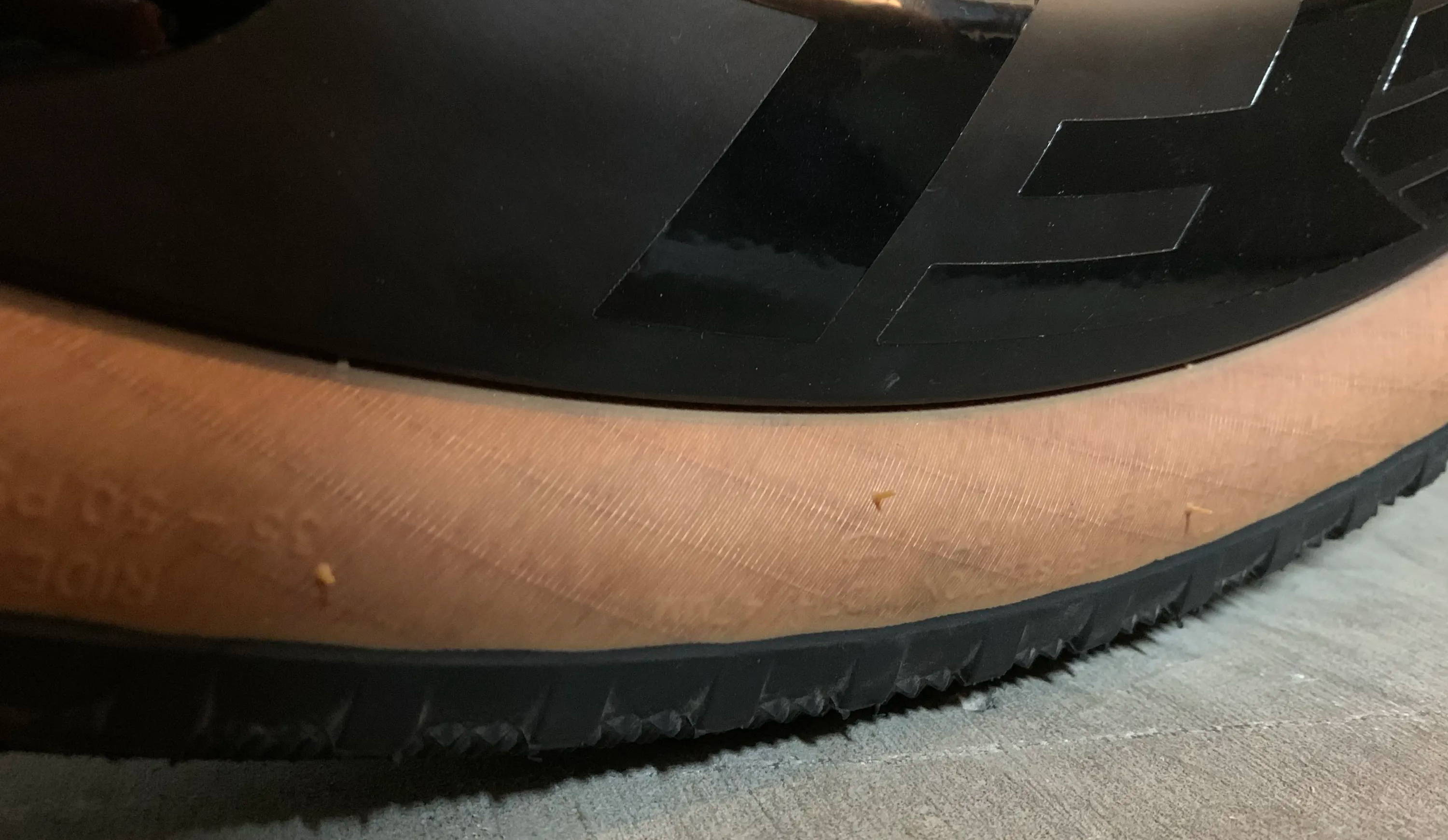# Aerodynamics And Rolling Resistance At Different Rider Speeds

I was recently working on a problem to determine the number of watts required to overcome aerodynamic drag and rolling resistance at different rider speeds. What I found surprised me. While I know aerodynamics grows quickly, I didn’t know how quickly.

Our FLO All Sport and Gravel wheels were designed to optimize both aerodynamic drag and rolling resistance. The resulting products are the fastest we’ve ever made. To show the significance of aerodynamic drag and rolling resistance to you, a cyclist, I wrote this article which walks through the math and the importance each is to your overall speed.# The Chung Method

For starters, let’s refer to the great work by Robert Chung. The Chung Method breaks down the number of watts required to propel a bike forward at a specific ground speed.

This equation can be broken down into four parts.

This gives us the equation of:

This can be further broken down to:

Each variable of the equation above is listed below:

v = speed in m/s (i.e., “ground” speed)
m = total mass (kg) of rider + bike
g = 9.81 m/sec2
Crr = coefficient of rolling resistance
s = slope
a = acceleration
ρ = air density
v = “air” speed of bike air
CdA = drag area

# Assumptions For Our Example

To make things a bit easier to understand we are going to assume that the slope is 0 degrees and that our acceleration is 0. This will allow us to simplify the equation to the following:

Using this equation, we can see that if given a ground speed and an air speed, we can determine the total watts required to ride at that speed. We can also see how many watts account for aerodynamics and rolling resistance.

In this example, we are going to assume that the wind speed is 0 which means our Vair is going to equal the ground speed. We will also assume the following about our rider so we can calculate the watt value.

Crr = 0.0026
m = 81 kg
v = 10 mph or 4.4704 m/s
CdA = 0.35
ρ = 1.225

# Results From 1-30 Miles Per Hour

Now that we understand how the equation works, the table below shows the watt values required to be overcome for speed from 1 to 30 mph. Notice how rolling resistance starts higher, the two meet around 10mph, and then aerodynamic drag takes off quickly. This is because of the cubic factor of the velocity component of the aerodynamic drag.

# Final Thoughts

As you can see, both aerodynamics and rolling resistance are important when you are trying to improve your overall speed. We can see that the faster you go, the more it matters. In a few weeks we will look deeper into rolling resistance and how it is affected by speed when the Crr value changes. This will highlight the importance of a wheel that lowers your rolling resistance like the FLO All Sport and Gravel Wheels. After, we will cover the aerodynamic component in more detail.

Let us know your thoughts on how rolling resistance and aerodynamics affect your total watt output.